§ 3    Affine Coordinate System

1. Affine coordinate system and metric coefficients

[ Affine coordinates ]   In the three-dimensional Euclidean space V , if a rectangular coordinate system is taken, and its coordinate unit vectors are i , j , and k , the vector a in the space can be expressed as

a = a x i + a y   j + a z k

Generally, given three non-coplanar vectors e 1 , e 2 , e 3 in the space, any vector a in the space can be decomposed according to these three vectors, and let its coefficients be a 1 , a 2 , a 3 ( here 1 , 2, 3 are not exponents, but superscripts ) , then a can be expressed as

a = a 1 e 1 + a 2 e 2 + a 3 e 3

Or simply count as V V a = a i e i

a = a 1 , a 2 , a 3 ={ a i } V V V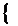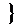Such coordinate systems e 1 , e 2 , e 3 are called affine coordinate systems, e 1 , e 2 , e 3 are called coordinate vectors, and a 1 , a 2 , a 3 are called affine coordinates of vector a .[ Metric coefficient in Euclidean space ]   When the vector a is written in the above form, its length a is given by

( a ) 2 = ( a i e i )( a j e j ) = ( e i e j ) a i a j

give . order

e i e j = g ij (= g ji )   ( i , j =1,2,3)

Then gij is called the metric coefficient of the affine coordinate system .

1 The length of the vector a is given by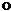( a ) 2 = g ij a i a j

Calculate .

2two vectors

a = a i e i , b = b j e j

The included angle is given by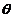cos=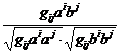Calculate .

3Since g ij a i a j is a positive definite quadratic form, the determinant made by g ij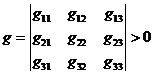mixed product

( e 1 , e 2 , e 3 ) 2 = = g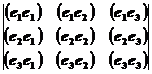( e 1 , e 2 , e 3 )=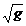[ Kronecker notation ]   Symmetric matrix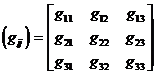The inverse matrix of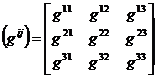to represent . By the properties of the inverse matrix, there are g ij = g ji and

g ik g kj =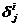in the formula=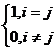called Kronecker notation .

[ reciprocal vector ]   use this g ij provision

e i = g ij e j

Hence there is

e j = g ij e i

e i e k =( g ij e j ) e k = g ij ( e j e k )= g ij g jk =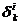e i e j =( g il e l )( g jm e m )= g il g jm ( e l e m )= g il g jm g lm = g il = g ij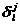For e 1 , e 2 , e 3 , we can get

e 1 =( e 2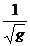× e 3 ),

e 2 =( e 3× e 1 ), e 3 =( e 1× e 2 )

e 1 , e 2 , e 3 are called reciprocal vectorsabout the coordinate vectors e 1 , e 2 , e 3. g ij is called the metric coefficient inthe affine coordinate system of the reciprocal vectors.

Two, contravariant vector and covariant vector

[ Contravariant vector and covariant vector ]   If the affine coordinates a 1 , a 2 , a 3 of the vector a in the coordinate systems e 1 , e 2 , e 3 are determined by the formulaa = a 1 e 1 + a 2 e 2 + a 3 e 3 = a i e i

Given, a 1 , a 2 , a 3 are called contravariant coordinates ( or called anti-variation coordinates ) of vector a , and vector a i is called a contravariant vector ( or called anti-variation vector ).If the reciprocal vectors about the coordinate vectors e 1 , e 2 , e 3 are e 1 , e 2 , e 3 , the affine coordinates a 1 , a 2 of the vector a in the coordinate systems e 1 , e 2 , e 3 , a3 is determined by the formulaa = a 1 e 1 + a 2 e 2 + a 3 e 3 = a j e j

given, then a 1 , a 2 , a 3 are called covariant coordinates of vector a, and vector a j is called covariant vector .In the Cartesian coordinate system, the covariant coordinates and contravariant coordinates of the vector are consistent . Generally, in the affine coordinate system, the covariant coordinates and the contravariant coordinates have a relationship

a i = a · e i =( a j e j ) · e i = a j ( e j · e i )= a j g ji

[ scalar product of contravariant vector and covariant vector ]

If a , b are two vectors, a 1 , a 2 , a 3 ; b 1 , b 2 , b 3 are their contravariant coordinates, respectively, then

a · b = g ij a i b j

If a , b are two vectors, a 1 , a 2 , a 3 ; b 1 , b 2 , b 3 are their covariant coordinates, respectively, then

a · b = g ij a i a j

If the contravariant coordinates of a are a 1 , a 2 , a 3 , and the covariant coordinates of b are b 1 , b 2 , b 3 , then

a · b = a i b i

Three, n -dimensional space

[ Definition of n -dimensional space ]   If a point in the space has a one-to-one correspondence with the values ​​of an ordered group of n independent real numbers x 1 , ···, x n , then, take such a point as an element The set of is called n -dimensional real number space V ( referred to as n -dimensional space ) , denoted as R n . So a point M in the space corresponds to a set of ordered numbers x 1 ,..., x n ; on the contrary, a set of ordered numbers x 1 , ···, x n corresponds to a point M. Such a set of ordered numbers ( x 1 , ···, x n ) is called an n -dimensional spaceThe coordinates of a point M in R n .

[ Vector in n -dimensional space ]   Take a certain point O in the n -dimensional space R n with coordinates (0,0, ··· ,0) , and another point M ( x 1 , x 2 , ···, x n ) , r is a vector corresponding to two points O and M , called the vector radius of point M.

It is assumed that an affine coordinate system can be introduced in R n such that the relationship between the vector radius r and the coordinates of the point M ( x i ) is

r = x 1 e 1 +... + x n e n = x i e i

where e 1 , ···, e n are n linearly independent vectors in R n , and this coordinate system e 1 , ··· , e n is called an affine coordinate system in R n , x 1 , · , x n is called the affine coordinate of the vector r in R n .Many of the results discussed in the three-dimensional space are valid in the n -dimensional space, as long as the indicators appearing in the formula are considered to be from 1 to n .

[ Contravariant vector and covariant vector ]   Consider an arbitrary coordinate transformation in the n -dimensional space R n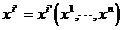V V (1)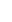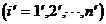where the function has successive derivatives with respect to x i ( the order required in the discussion ) , and the Jacobian of the transformation is not equal to zero: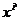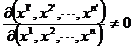Therefore (1) has an inverse transform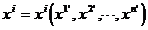Let a 1 , ··· , a n be the function of x i , if under the coordinate transformation, they are all transformed according to the coordinate differential, that is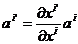Then a i is called the contravariant coordinate of a vector in the coordinate system ( x i ) , and it is the contravariant coordinate of the same vector in the coordinate system . The vector is called the contravariant vector .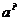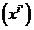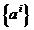If a i press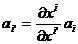form transformation, then a i is called the covariant coordinate of a vector in the coordinate system ( x i ) , and it is called the covariant coordinate of the same vector in the coordinate system, and the vector is called the covariant vector .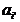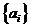The transformation coefficients of contravariant and covariant vectors are different, but there is a relation between them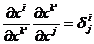where is the Kronecker notation .The gradient of a scalar field is a covariant vector .

Let the scalar field in n -dimensional space be , its change along an infinitesimal displacement d x i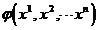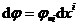is an invariant under the coordinate transformation, where is the component of the gradient . Therefore, under the coordinate transformation,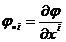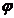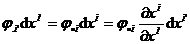but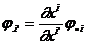So it is a covariant vector .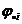V Euclidean space is abbreviated as Euclidean space, and its definition can be found in Chapter 21, §4.

The abbreviation V V is the way it is written in tensor arithmetic.If each indicator appears once in the product, it means it takes all possible values; if

Each indicator appears twice in the product, which means that all possible values ​​are taken, and then the items are added together to find the sum . This rule is called

Agreement for Einstein .

V V V This is the tensor notation.

For another definition of V n -dimensional real number space, see Chapter 21,§3.

V V is used here torepresent the coordinates of the same point M ( xi ) in another coordinate system, that is to say,andsamepoint.

A kernel character ( such as x ) represents the same object, and a prime is added to the index to represent different coordinate systems (such as etc.), this notation is called kernel

standard method .